Win up to 100% scholarship on Aakash BYJU'S JEE/NEET courses with ABNAT Win up to 100% scholarship on Aakash BYJU'S JEE/NEET courses with ABNAT

# JEE Main 2022 July 25 – Shift 1 Physics Question Paper with Solutions

The JEE Main 2022 July 25 – Shift 1 Physics Question Paper with Solutions is given on this page. JEE Main 2022 question paper with solutions consists of accurate solutions, which helps the students to understand concepts clearly. BYJU’S provides clear and step-by-step solutions prepared by our subject matter experts. Students are recommended to practice JEE Main 2022 question papers so that they can improve their speed and accuracy. These solutions help to develop confidence among students who are preparing for exams. JEE Main 2022 answer keys can be used to check the correct answers to the exam.

## JEE Main 2022 July 25th Morning Shift Physics Question Paper and Solutions

SECTION – A

Multiple Choice Questions: This section contains 20 multiple choice questions. Each question has 4 choices (1), (2), (3) and (4), out of which ONLY ONE is correct.

1. If momentum [P], area [A] and time [T] are taken as fundamental quantities, then the dimensional formula for the coefficient of viscosity is

(A) [PA–1T0]

(B) [PAT–1]

(C) [PA–1T]

(D) [PA–1T–1]

Sol.

$$\begin{array}{l} \left[\eta \right]=\left[ML^{-1}T^{-1}\right]\end{array}$$

Now if

$$\begin{array}{l} \left[\eta \right]=\left[P\right]^a\left[A\right]^b\left[T\right]^c\end{array}$$
$$\begin{array}{l} \Rightarrow\ \left[ML^{-1}T^{-1}\right]=\left[ML^1T^{-1}\right]^a\left[L^2\right]^b\left[T\right]^c\end{array}$$

a = 1, a + 2b = – 1, – a + c = – 1

a = 1, b = –1, c = 0

⇒ [η] = [P] [A]–1 [T]0

= [PA–1T0]

2. Which of the following physical quantities have the same dimensions?

$$\begin{array}{l}(A)\ \text{Electric displacement}\left(\overrightarrow{D}\right)\ and\ \text{surface charge density}\end{array}$$

(B) Displacement current and electric field

(C) Current density and surface charge density

(D) Electric potential and energy

Sol.

$$\begin{array}{l}\text{Electric displacement } \left(\overrightarrow{D}\right)=\varepsilon_0\overrightarrow{E}\end{array}$$
$$\begin{array}{l} \Rightarrow\ \left[\overrightarrow{D}\right] =\left[\varepsilon_0\right]\left[\overrightarrow{E}\right]\end{array}$$
$$\begin{array}{l}=\left[M^{–1}L^{–3}T^4A^2\right]\left[M^{1}L^{1}A^{–1}T^{–3}\right]\end{array}$$
$$\begin{array}{l} \left[\overrightarrow{D}\right]=\left[L^{-2}T^1A^1\right]\end{array}$$
$$\begin{array}{l}\text{[Surface charge density]} =\frac{\left[Q\right]}{\left[A\right]} \end{array}$$
[σ] = [ATL–2]
$$\begin{array}{l}\Rightarrow \overrightarrow{D}\ \text{and}\ [\sigma\ \text{have same dimensions}]\end{array}$$

3. A person moved from A to B on a circular path as shown in figure. If the distance travelled by him is 60 m, then the magnitude of displacement would be

(Given cos135°= –0.7)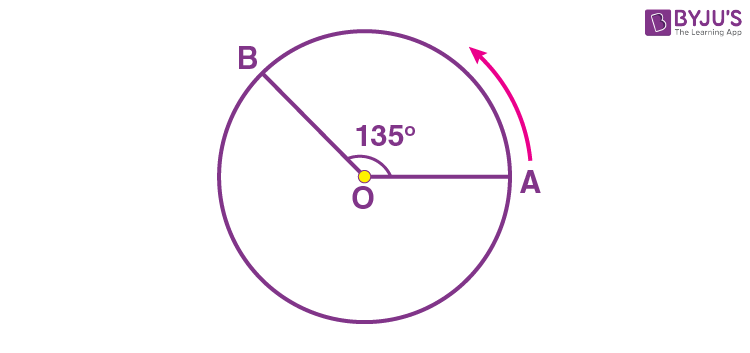(A) 42 m

(B) 47 m

(C) 19 m

(D) 40 m

Sol. Distance travelled = 60 m

⇒ Angle covered = 135°

$$\begin{array}{l} \text{Displacement}=2R\sin\left(\frac{135^\circ}{2}\right)\end{array}$$
$$\begin{array}{l} =2\left(\dfrac{60}{135}\times \frac{180}{\pi}\right)\left[\frac{1-\cos\left(135^\circ\right)}{2}\right]^{1/2}\end{array}$$
$$\begin{array}{l} =2\left(\frac{80}{\pi}\right)\left(0.85\right)^{1/2}\approx 47\end{array}$$

4. A body of mass 0.5 kg travels on straight line path with velocity v = (3x2 + 4) m/s. The network done by the force during its displacement from x = 0 to x = 2 m is

(A) 64 J

(B) 60 J

(C) 120 J

(D) 128 J

Sol.

$$\begin{array}{l} v = 3x^2 + 4\end{array}$$

at x = 0, v1 = 4 m/s

x = 2, v2 = 16 m/s

$$\begin{array}{l}\Rightarrow \text{Work done} = \triangle \text{kinetic energy} \end{array}$$
$$\begin{array}{l} =\frac{1}{2}\times m\left(v_2^2-v_1^2\right) \end{array}$$
$$\begin{array}{l} =\frac{1}{4} \left(256-16\right)= 60 ~\text{J}\end{array}$$

5. A solid cylinder and a solid sphere, having same mass M and radius R, roll down the same inclined plane from top without slipping. They start from rest. The ratio of velocity of the solid cylinder to that of the solid sphere, with which they reach the ground, will be

$$\begin{array}{l} (\text{A})\sqrt{\frac{5}{3}} \end{array}$$
$$\begin{array}{l} (\text{B})\sqrt{\frac{4}{5}} \end{array}$$
$$\begin{array}{l} (\text{C})\sqrt{\frac{3}{5}} \end{array}$$
$$\begin{array}{l} (\text{D})\sqrt{\frac{14}{15}} \end{array}$$

Sol.

$$\begin{array}{l} a=\frac{g\sin\theta}{1+\frac{K^2}{R^2}}\end{array}$$
$$\begin{array}{l} v=\sqrt{\frac{2Sg\sin\theta}{1+\frac{K^2}{R^2}}}\end{array}$$
$$\begin{array}{l} \Rightarrow\ \frac{v_c}{v_{ss}}\sqrt{\frac{1+\frac{K_{ss}^2}{R^2}}{1+\frac{K_c^2}{R^2}}}=\sqrt{\frac{1+\frac{2}{5}}{1+\frac{1}{2}}}\end{array}$$
$$\begin{array}{l} \Rightarrow\ \sqrt{\frac{\frac{7}{5}}{\frac{3}{2}}}=\sqrt{\frac{14}{15}}\end{array}$$

6. Three identical particles A, B and C of mass 100 kg each are placed in a straight line with AB = BC = 13 m. The gravitational force on a fourth particle P of the same mass is F, when placed at a distance 13 m from the particle B on the perpendicular bisector of the line AC. The value of F will be approximately

(A) 21G

(B) 100G

(C) 59G

(D) 42G

Sol.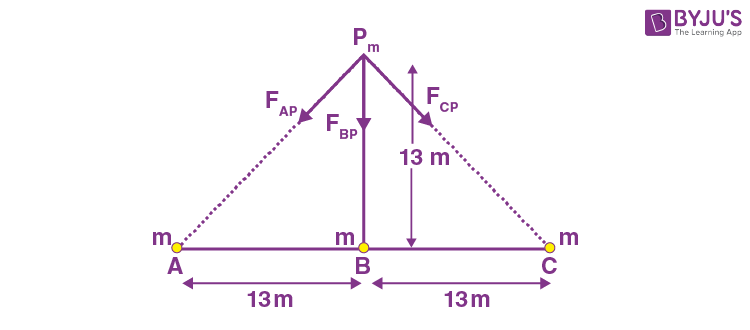$$\begin{array}{l}\text{m} = 100 ~\text{kg}\end{array}$$

$$\begin{array}{l} F_{AP}=\frac{Gm^2}{\left(13\sqrt{2}\right)^2} \end{array}$$
$$\begin{array}{l} F_{BP}=\frac{Gm^2}{13^2} \end{array}$$
$$\begin{array}{l} F_{CP}=\frac{Gm^2}{\left(13\sqrt{2}\right)^2} \end{array}$$
$$\begin{array}{l}F_{net} = F_{BP} + F_{AP}~cos45^\circ + F_{CP}~cos45^\circ \end{array}$$
$$\begin{array}{l} =\frac{Gm^2}{13^2}\left(1+\frac{1}{\sqrt{2}}\right)\end{array}$$
$$\begin{array}{l} =\frac{G100^2}{169}\left(1+0.707\right)\simeq 100G\end{array}$$

7. A certain amount of gas of volume V at 27°C temperature and pressure 2 × 107 Nm–2 expands isothermally until its volume gets doubled. Later it expands adiabatically until its volume gets redoubled. The final pressure of the gas will be (Use, γ = 1.5)

$$\begin{array}{l}(A)\ 3.536 \times 10^5 ~\text{Pa}\end{array}$$
$$\begin{array}{l}(B)\ 3.536 \times 10^6 ~\text{Pa}\end{array}$$
$$\begin{array}{l}(C)\ 1.25 \times 10^6 ~\text{Pa}\end{array}$$
$$\begin{array}{l}(D)\ 1.25 \times 10^5 ~\text{Pa}\end{array}$$

Sol.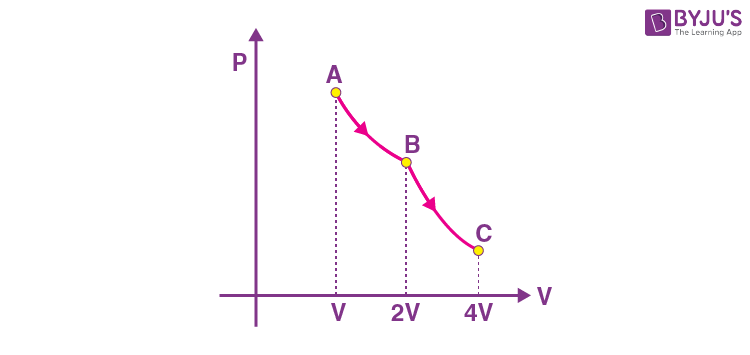Let AB is isothermal process and BC is adiabatic process, then for AB process

$$\begin{array}{l}P_AV_A= P_BV_B\end{array}$$

$$\begin{array}{l}\Rightarrow P_B= 10^7~\text{Nm}^{-2}\end{array}$$

For process BC

$$\begin{array}{l} P_BV_B^r =P_CV_C^r\end{array}$$
$$\begin{array}{l}P_C = 3.536~x \times 10^6~\text{Pa}\end{array}$$

8. Following statements are given:

(A) The average kinetic energy of a gas molecule decreases when the temperature is reduced.

(B) The average kinetic energy of a gas molecule increases with increase in pressure at constant temperature.

(C) The average kinetic energy of a gas molecule decreases with increase in volume.

(D) Pressure of a gas increases with increase in temperature at constant pressure.

(E) The volume of gas decreases with increase in temperature.

Choose the correct answer from the options given below:

(A) (A) & (D) only

(B) (A), (B) & (D) only

(C) (B) & (D) only

(D) (A), (B) & (E) only

Sol. Because KE ∝T so A is correct, B is incorrect, statement C cannot be said, statement D is contradicting itself, statement E is incorrect (Isothermal process)

If the statement of D would have been.

“Pressure of gas increases with increase in temperature at constant volume, “then statement D would have been correct, so in that case answer would have been ‘A’.

9. In figure (A), mass ‘2 m‘ is fixed on mass ‘m‘, which is attached to two springs of spring constant k. In figure (B), mass ‘m‘ is attached to two springs of spring constant ‘k‘ and ‘2k‘. If mass ‘m‘ in (A) and in (B) are displaced by distance’ x’ horizontally and then released, then time period T1 and T2 corresponding to (A) and (B) respectively follow the relation.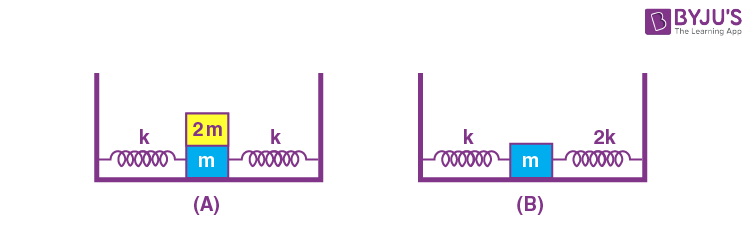$$\begin{array}{l} (\text{A})\ \frac{T_1}{T_2}=\frac{3}{\sqrt{2}}\end{array}$$
$$\begin{array}{l} (\text{B})\ \frac{T_1}{T_2}=\sqrt{\frac{3}{2}}\end{array}$$
$$\begin{array}{l} (\text{C})\ \frac{T_1}{T_2}=\sqrt{\frac{2}{3}}\end{array}$$
$$\begin{array}{l} (\text{D})\ \frac{T_1}{T_2}=\frac{\sqrt{2}}{3}\end{array}$$

Sol. Both the springs are in parallel combination in both the diagrams so

$$\begin{array}{l} T_1=2\pi\sqrt{\frac{3m}{2k}}\end{array}$$
$$\begin{array}{l} \text{and }T_2=2\pi\sqrt{\frac{m}{3k}}\end{array}$$
$$\begin{array}{l} \text{So, }\frac{T_1}{T_2}=\frac{3}{\sqrt{2}}\end{array}$$

10. A condenser of 2μF capacitance is charged steadily from 0 to 5 C. Which of the following graph represents correctly the variation of potential difference (V) across it’s plates with respect to the charge (Q) on the condenser?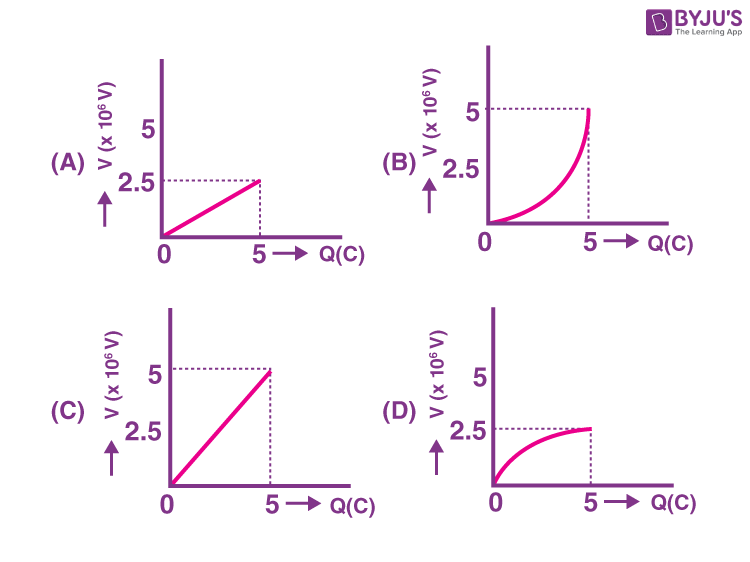Sol.

$$\begin{array}{l}Q=CV \\\text{As capacitance is constant}\ Q\propto V\end{array}$$
$$\begin{array}{l} \text{and}~V_f=\frac{Q_f}{C}=\frac{5}{2\times10^{-6}}=2.5\times10^6~\text{V} \end{array}$$

So correct graph will be A.

11. Two charged particles, having same kinetic energy, are allowed to pass through a uniform magnetic field perpendicular to the direction of motion. If the ratio of radii of their circular path is 6 : 5 and their respective masses ratio is 9 : 4. Then, the ratio of their charges will be :

(A) 8 : 5

(B) 5 : 4

(C) 5 : 3

(D) 8 : 7

Sol.

$$\begin{array}{l}\text{We know that}\ R=\frac{mv}{Bq}=\sqrt{\frac{2mk}{Bq}} \end{array}$$
$$\begin{array}{l}\Rightarrow \text{Ratio of radii}=\frac{R_1}{R_2}=\sqrt{\frac{m_1}{m_2}}\frac{q_2}{q_1}\end{array}$$
$$\begin{array}{l} \Rightarrow\ \frac{6}{5}=\sqrt{\frac{9}{4}}\frac{q_2}{q_1} \end{array}$$
$$\begin{array}{l} \Rightarrow\ \frac{q_1}{q_2}=\frac{3}{2}\times\frac{5}{6}=\frac{5}{4}\end{array}$$

12. To increase the resonant frequency in series LCR circuit,

(A) Source frequency should be increased.

(B) Another resistance should be added in series with the first resistance.

(C) Another capacitor should be added in series with the first capacitor.

(D) The source frequency should be decreased.

Sol.

$$\begin{array}{l} \text{Resonant frequency}=\frac{1}{\sqrt{LC}}=\omega_0\end{array}$$
$$\begin{array}{l}\Rightarrow \text{If we decrease}~C,\omega_0~ \text{would increase} \end{array}$$

⇒Another capacitor should be added in series.

13. A small square loop of wire of side l is placed inside a large square loop of wire L(L>>l). Both loops are coplanar and their centres coincide at point O as shown in figure. The mutual inductance of the system is :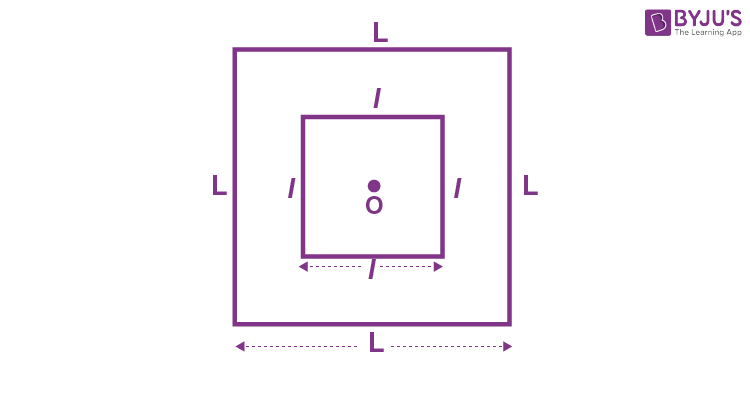$$\begin{array}{l} (\text{A})\ \frac{2\sqrt{2}\mu_0L^2}{\pi I}\end{array}$$
$$\begin{array}{l} (\text{B})\ \frac{\mu_0I^2}{2\sqrt{2}\ \pi L}\end{array}$$
$$\begin{array}{l} (\text{C})\ \frac{2\sqrt{2}\mu_0I^2}{\pi L}\end{array}$$
$$\begin{array}{l} (\text{D})\ \frac{\mu_0L^2}{2\sqrt{2}\pi I}\end{array}$$

Sol.

$$\begin{array}{l} \text{We know}~\phi = Mi\end{array}$$

Let i current be flowing in the larger loop

$$\begin{array}{l} \Rightarrow \phi\simeq \left[4\times\frac{\mu_0i}{4\pi\left(L/2\right)}\left[\sin 45^\circ+\sin 45^\circ\right]\right]\times\text{Area}\end{array}$$
$$\begin{array}{l} =\frac{2\sqrt{2}\mu_0i}{\pi L}\times I^2 \end{array}$$
$$\begin{array}{l} \Rightarrow M=\frac{\phi}{i}=\frac{2\sqrt{2}\mu_0I^2}{\pi L}\end{array}$$

14. The rms value of conduction current in a parallel plate capacitor is 6.9 μA. The capacity of this capacitor, if it is connected to 230 V ac supply with an angular frequency of 600 rad/s, will be :

(A) 5 pF

(B) 50 pF

(C) 100 pF

(D) 200 pF

Sol.

$$\begin{array}{l} Z_c=\frac{V}{I} \end{array}$$
$$\begin{array}{l} \Rightarrow \frac{1}{\omega C}=\frac{230}{6.9}M\Omega\end{array}$$
$$\begin{array}{l}\Rightarrow C=\frac{6.9}{230\omega} \mu \text{F}\end{array}$$
$$\begin{array}{l} =\frac{6.9}{230\times 600}\mu \text{F} \\C = 50 pF\end{array}$$

15. Which of the following statement is correct?

(A) In primary rainbow, observer sees red colour on the top and violet on the bottom

(B) In primary rainbow, observer sees violet colour on the top and red on the bottom

(C) In primary rainbow, light wave suffers total internal reflection twice before coming out of water drops

(D) Primary rainbow is less bright than secondary rainbow

Sol. In primary rainbow, observer sees red colour on the top and violet on the bottom.

16. Time taken by light to travel in two different materials A and B of refractive indices μA and μB of same thickness is t1 and t2 respectively. If t2t1 = 5 × 10–10 s and the ratio of μA to μB is 1 : 2. Then, the thickness of material, in meter is: (Given vA and vB are velocities of light in A and B materials, respectively.)

(A) 5 × 10-10 vA m

(B) 5 × 10-10 m

(C) 1.5 × 10-10 m

(D) 5 × 10-10 vB m

Sol.

$$\begin{array}{l}t_2-t_1=5\times10^{-10}\end{array}$$
$$\begin{array}{l} \Rightarrow\frac{d}{v_B}-\frac{d}{v_A}=5\times 10^{-10}\end{array}$$

and,

$$\begin{array}{l} \frac{v_B}{v_A}=\frac{\mu_A}{\mu_B}=\frac{1}{2}\end{array}$$
$$\begin{array}{l} \Rightarrow d\left(1-\frac{v_B}{v_A}\right)=5\times 10^{-10} \times v_B\end{array}$$
$$\begin{array}{l} \Rightarrow d\left(1-\frac{1}{2}\right)=5\times 10^{-10}\times v_B\end{array}$$
$$\begin{array}{l}\Rightarrow d = 10 \times 10^{-10}\times v_Bm\end{array}$$
$$\begin{array}{l}\Rightarrow d = 5\times 10^{-10}\times v_A \text{m}\end{array}$$

17. A metal exposed to light of wavelength 800 nm and emits photoelectrons with a certain kinetic energy. The maximum kinetic energy of photo-electron doubles when light of wavelength 500 nm is used. The work function of the metal is:

(Take hc = 1230 eV-nm)

(A) 1.537 eV

(B) 2.46 eV

(C) 0.615 eV

(D) 1.23 eV

Sol.

$$\begin{array}{l} \because K_m=\frac{hc}{\lambda}-\phi\end{array}$$
$$\begin{array}{l} \Rightarrow K=\frac{1230}{800}-\phi\end{array}$$

and,

$$\begin{array}{l} 2K=\frac{1230}{500}-\phi\end{array}$$
$$\begin{array}{l} \Rightarrow 2\times \frac{1230}{800}-2\phi = \frac{1230}{500}-\phi \\\Rightarrow \phi = 0.615\ eV\end{array}$$

18. The momentum of an electron revolving in nth orbit is given by: (Symbols have their usual meanings)

$$\begin{array}{l} (\text{A})\ \frac{nh}{2\pi r}\end{array}$$
$$\begin{array}{l} (\text{B})\ \frac{nh}{2r}\end{array}$$
$$\begin{array}{l} (\text{C})\ \frac{nh}{2\pi}\end{array}$$
$$\begin{array}{l} (\text{D})\ \frac{2\pi r}{nh}\end{array}$$

Sol.

$$\begin{array}{l} \because mvr=\frac{nh}{2\pi}\end{array}$$
$$\begin{array}{l} \Rightarrow mv=\frac{nh}{2\pi r}\end{array}$$

19. The magnetic moment of an electron (e) revolving in an orbit around nucleus with an orbital angular momentum is given by:

$$\begin{array}{l} (\text{A})\ \overrightarrow{\mu_L}=\frac{\overrightarrow{eL}}{2m}\end{array}$$
$$\begin{array}{l} (\text{B})\ \overrightarrow{\mu_L}=-\frac{\overrightarrow{eL}}{2m}\end{array}$$
$$\begin{array}{l} (\text{C})\ \overrightarrow{\mu_I}=-\frac{\overrightarrow{eL}}{m}\end{array}$$
$$\begin{array}{l} (\text{D})\ \overrightarrow{\mu_I}=\frac{2\overrightarrow{eL}}{m}\end{array}$$

Sol.

$$\begin{array}{l} \because \overrightarrow{\mu}=\frac{q\overrightarrow{L}}{2m} \end{array}$$
$$\begin{array}{l} \Rightarrow \overrightarrow{\mu}=\frac{-e\overrightarrow{L}}{2m} \end{array}$$

20. In the circuit, the logical value of A = 1 or B = 1 when potential at A or B is 5 V and the logical value of
A = 0 or B = 0 when potential at A or B is 0 V.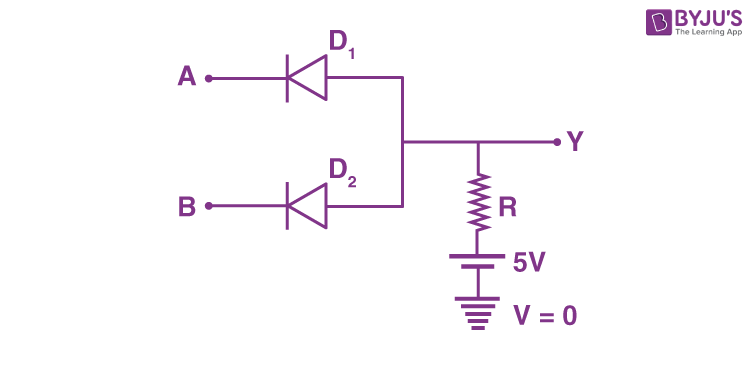The truth table of the given circuit will be:

(A) A B Y

0 0 0

1 0 0

0 1 0

1 1 1

(B) A B Y

0 0 0

1 0 1

0 1 1

1 1 1

(C) A B Y

0 0 0

1 0 0

0 1 0

1 1 0

(D) A B Y

0 0 1

1 0 1

0 1 1

1 1 0

Sol. Given circuit is equivalent to an AND gate.

∴ A B Y

0 0 0

0 1 0

1 0 0

1 1 1

SECTION – B

Numerical Value Type Questions: This section contains 10 questions. In Section B, attempt any five questions out of 10. The answer to each question is a NUMERICAL VALUE. For each question, enter the correct numerical value (in decimal notation, truncated/rounded-off to the second decimal place; e.g. 06.25, 07.00, –00.33, –00.30, 30.27, –27.30) using the mouse and the on-screen virtual numeric keypad in the place designated to enter the answer.

1. A car is moving with speed of 150 km/h and after applying the break it will move 27 m before it stops. If the same car is moving with a speed of one third the reported speed then it will stop after travelling ___ m distance.

Sol.

$$\begin{array}{l} F_Rd=\frac{1}{2}mv^2\\\frac{d_2}{d_1}=\left(\frac{v_2}{v_1}\right)^2=\left(\frac{1}{3}\right)^2\\ d_2=d_1\times\frac{1}{9}=3m\end{array}$$

2. For forces are acting at a point P in equilibrium as shown in figure. The ratio of force F1 to F2 is 1 : x where x =___.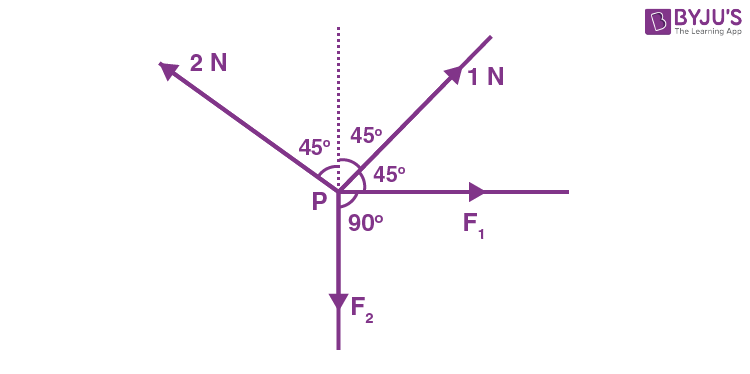Sol.

$$\begin{array}{l} F_1=+2\times\frac{1}{\sqrt{2}}-\frac{1}{\sqrt{2}}=\frac{1}{\sqrt{2}}\\F_2=2\times\frac{1}{\sqrt{2}}+\frac{1}{\sqrt{2}}=\frac{3}{\sqrt{2}}\end{array}$$$$\begin{array}{l} \frac{F_1}{F_2}=\frac{1}{3}=\frac{1}{x}\Rightarrow x=3\end{array}$$

3. A wire of length L and radius r is clamped rigidly at one end. When the other end of the wire is pulled by a force F, its length increases by 5 cm. Another wire of the same material of length 4L and radius 4r is pulled by a force 4F under same conditions. The increase in length of this wire is ___ cm.

Sol.

$$\begin{array}{l} \frac{F/A}{\Delta L/L}=Y\\ \Rightarrow \Delta L = \frac{FL}{AY}\\\frac{\Delta L_2}{\Delta L_1}=\left(\frac{F_2}{F_1}\right)\times\left(\frac{L_2}{L_1}\right)\times\left(\frac{A_1}{A_2}\right)\\ =4\times 4\times \frac{1}{16}=1\end{array}$$
$$\begin{array}{l}\Delta L_2 = \Delta L_1 = 5\text{ cm}\end{array}$$

4. A unit scale is to be prepared whose length does not change with temperature and remains 20 cm, using a bimetallic strip made of brass and iron each of different length. The length of both components would change in such a way that difference between their lengths remains constant. If length of brass is 40 cm and length of iron will be ___ cm.

$$\begin{array}{l}(\alpha_{iron} = 1.2 \times 10^{-5}K^{-1}~\text{and}~ \alpha_{brass} = 1.8 \times 10^{-5} K^{-1}).\end{array}$$

Sol.

$$\begin{array}{l}\Delta L_1 = \alpha_1L_1 \Delta T \\\Delta L_2 = \alpha_2L_2 \Delta T\end{array}$$
$$\begin{array}{l}\alpha_1L_1 = \alpha_2L_2 \\1.2 \times 10^{-5} \times L_1 = 1.8 \times 10^{-5}L_2\end{array}$$
$$\begin{array}{l}L_1=\frac{1.8}{1.2}\times40 = 60\ \text{ cm} \end{array}$$

5. An observer is riding on a bicycle and moving towards a hill at 18 kmh–1. He hears a sound from a source at some distance behind him directly as well as after its reflection from the hill. If the original frequency of the sound as emitted by source is 640 Hz and velocity of the sound in air is 320 m/s, the beat frequency between the two sounds heard by observer will be ___ Hz.

Sol.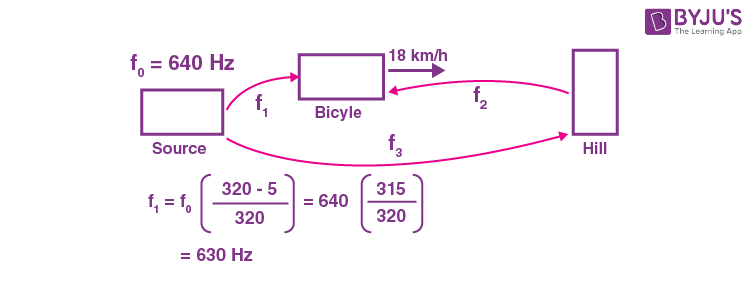$$\begin{array}{l}f_3 = f_0\text{ [No relative motion]}\end{array}$$
$$\begin{array}{l} f_2=f_0\left[\frac{320+5}{320}\right]=640\left(\frac{325}{320}\right)= 650\end{array}$$
$$\begin{array}{l}\text{Beat frequency} = f_2 – f_1 = 650 – 630 = 20 ~\text{Hz} \end{array}$$

6. The volume charge density of a sphere of radius 6 m is 2μC cm-3. The number of lines of force per unit surface area coming out from the surface of the sphere is _______ × 1010 NC-1.

$$\begin{array}{l}[Given \colon \text{ Permittivity of vacuum} \in_0 = 8.85 \times 10^{-12} C^2 N^{-1} – m^{-2}). \end{array}$$

Sol.

$$\begin{array}{l} \rho = 2 ~\mu \text{c/cm}^3\end{array}$$

R = 6 m

Number of lines of force per unit area = Electric field at surface.

$$\begin{array}{l} =\frac{KQ}{R^2} \end{array}$$
$$\begin{array}{l} =\frac{1}{4\pi\varepsilon_0}\frac{\rho\frac{4}{3}\pi R^3}{R^2}\end{array}$$
$$\begin{array}{l} =\frac{\rho R}{3\in_0} \end{array}$$
$$\begin{array}{l} =\frac{2\times 10^{-6}\times 10^6\times 6}{3\times 8.85\times 10^{-12}}\end{array}$$
$$\begin{array}{l}= 0.45197 \times 10^{12} \\= 45.19 \times 10^{10}\text{ N/C}\\\simeq 45 \times10^{10}\end{array}$$

7. In the given figure, the value of V0 will be ______ V.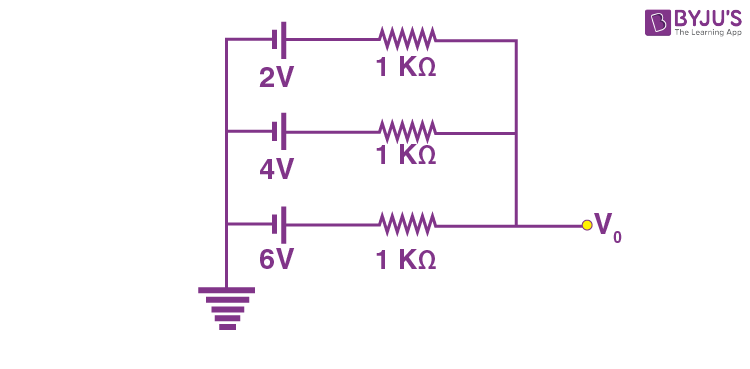Sol.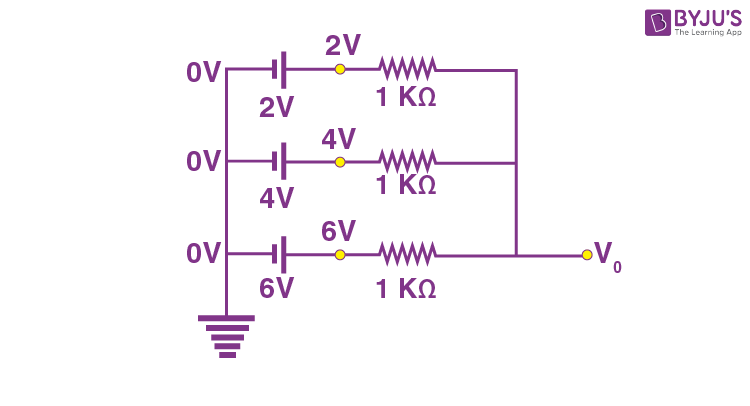Using Kirchhoff’s junction rule.

$$\begin{array}{l} \frac{2-V_0}{1}+\frac{4-V_0}{1}+\frac{6-V_0}{1}=0 \\12-3V_0=0\\V_0=4V\end{array}$$

8. Eight copper wire of length l and diameter d are joined in parallel to form a single composite conductor of resistance R. If a single copper wire of length 2l have the same resistance (R) then its diameter will be ________ d.

Sol.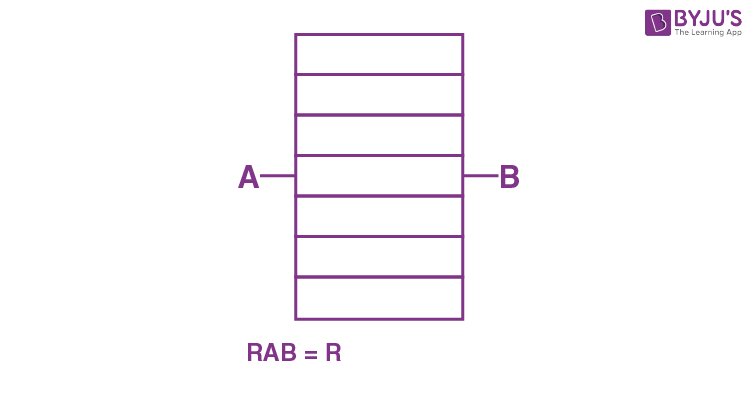$$\begin{array}{l} R=\frac{1}{8}\text{(Resistance of one wire)}\end{array}$$

$$\begin{array}{l} =\frac{1}{8}\rho \frac{I}{\pi\frac{d^2}{4}}=\frac{\rho I}{2\pi d^2} \end{array}$$

Resistance of copper wire of length 2l and diameter x = R.

$$\begin{array}{l} \rho \frac{2I}{\pi\frac{x^2}{4}}=R \end{array}$$
$$\begin{array}{l} \frac{8\rho I}{\pi X^2}=\frac{\rho I}{2\pi d^2}\\16d^2 = x^2\\x = 4d\end{array}$$

9. The energy band gap of semiconducting material to produce violet (wavelength = 4000 Å) LED is _________ eV. (Round off to the nearest integer).

Sol.

$$\begin{array}{l}\text{Energy corresponding to wavelength }4000\ AA \end{array}$$
$$\begin{array}{l} E=\frac{hc}{\lambda}\end{array}$$
$$\begin{array}{l} =\frac{6.6\times 10^{-34}\times 3 \times 10^8}{4000\times 10^{-10}\times1.6\times 10^{-19}}\text{eV} \end{array}$$
$$\begin{array}{l}=\frac{12400}{4000}\\= 3.1~\text{eV}\\\approx 3~\text{eV}\end{array}$$

10. The required height of a TV tower which can cover the population of 6.03 lakh is h. If the average population density is 100 per square km and the radius of earth is 6400 km, then the value of h will be ________ m.

Sol.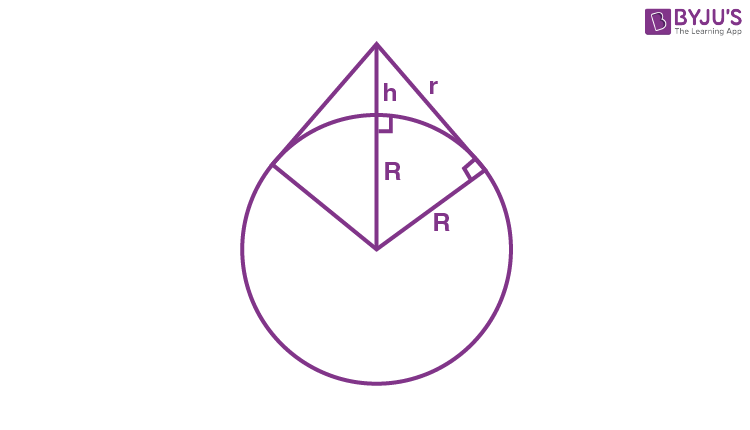$$\begin{array}{l} r=\sqrt{\left(h+R\right)^2-R^2}\cong \sqrt{2hR} \end{array}$$
$$\begin{array}{l} A=\frac{6.03\times 10^5}{100} \end{array}$$
$$\begin{array}{l}\pi r^2 = 6.03 \times 10^3\\ \pi2Rh = 6.03 \times 10^3\end{array}$$
$$\begin{array}{l} h=\frac{6.03\times 10^3}{2\times \pi \times R}=0.015\times 10\times 10^3 \text{ m}= 150 \text{ m}\end{array}$$

### Download PDF of JEE Main 2022 July 25 Shift 1 Physics Paper & Solutions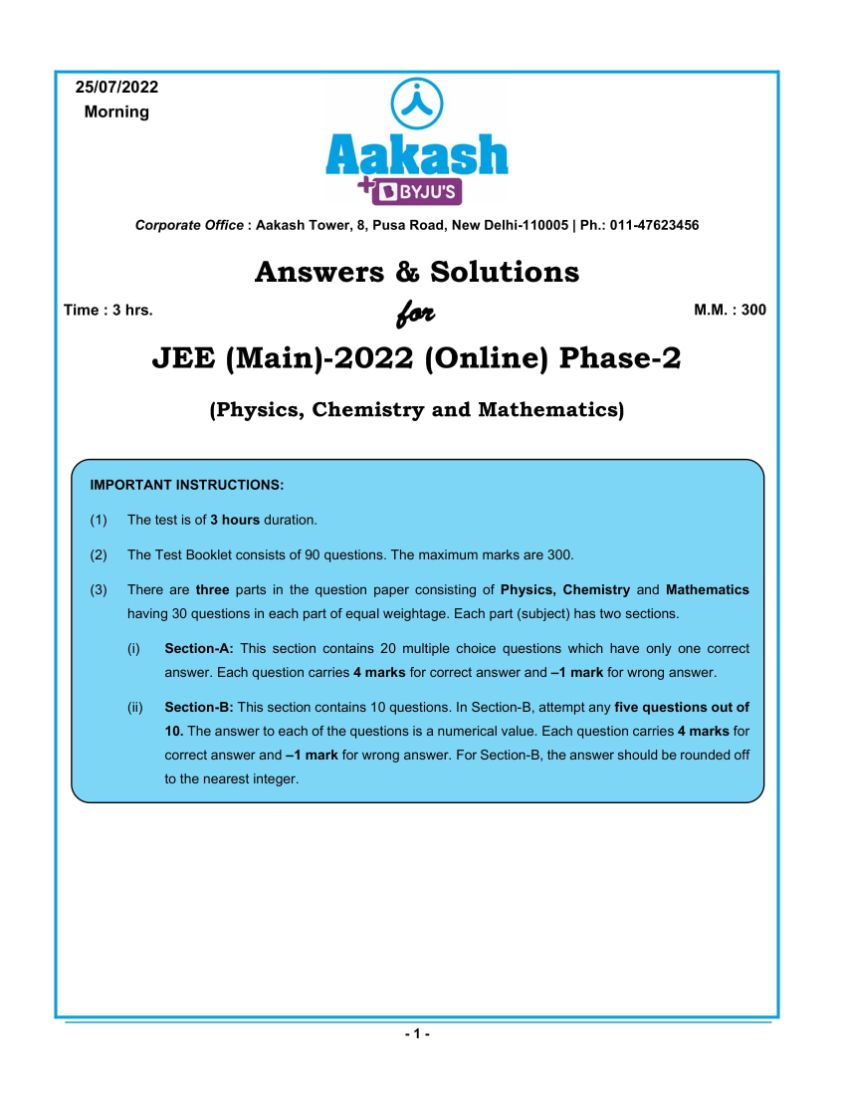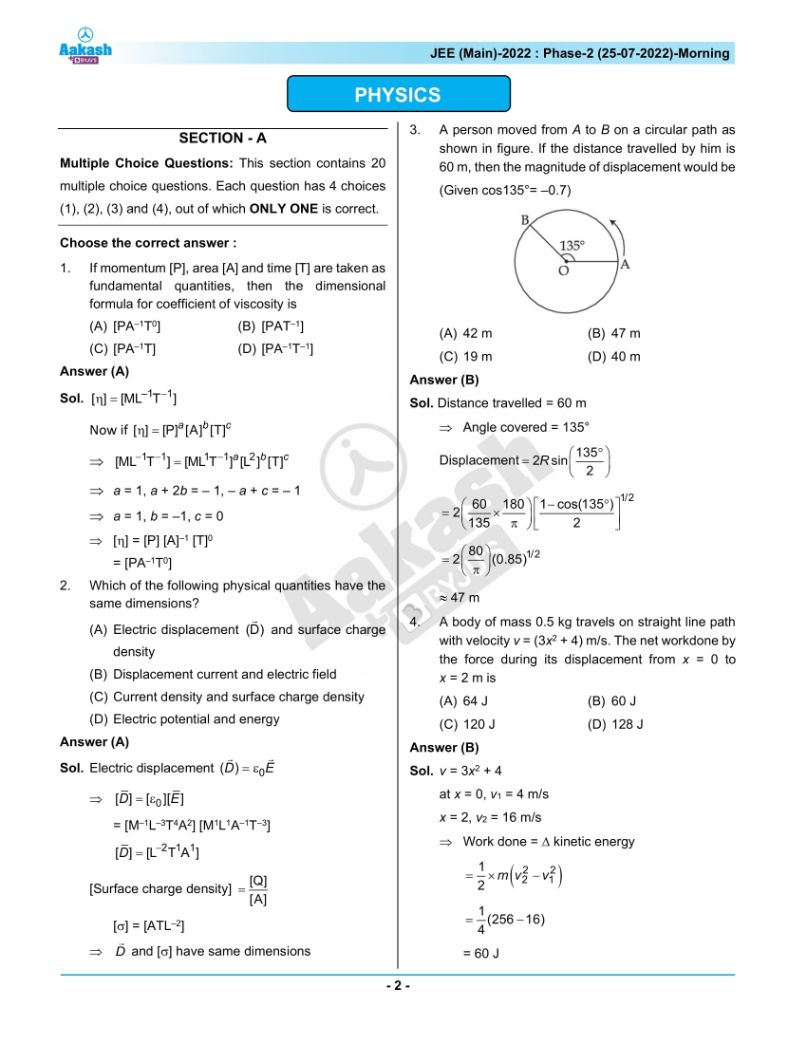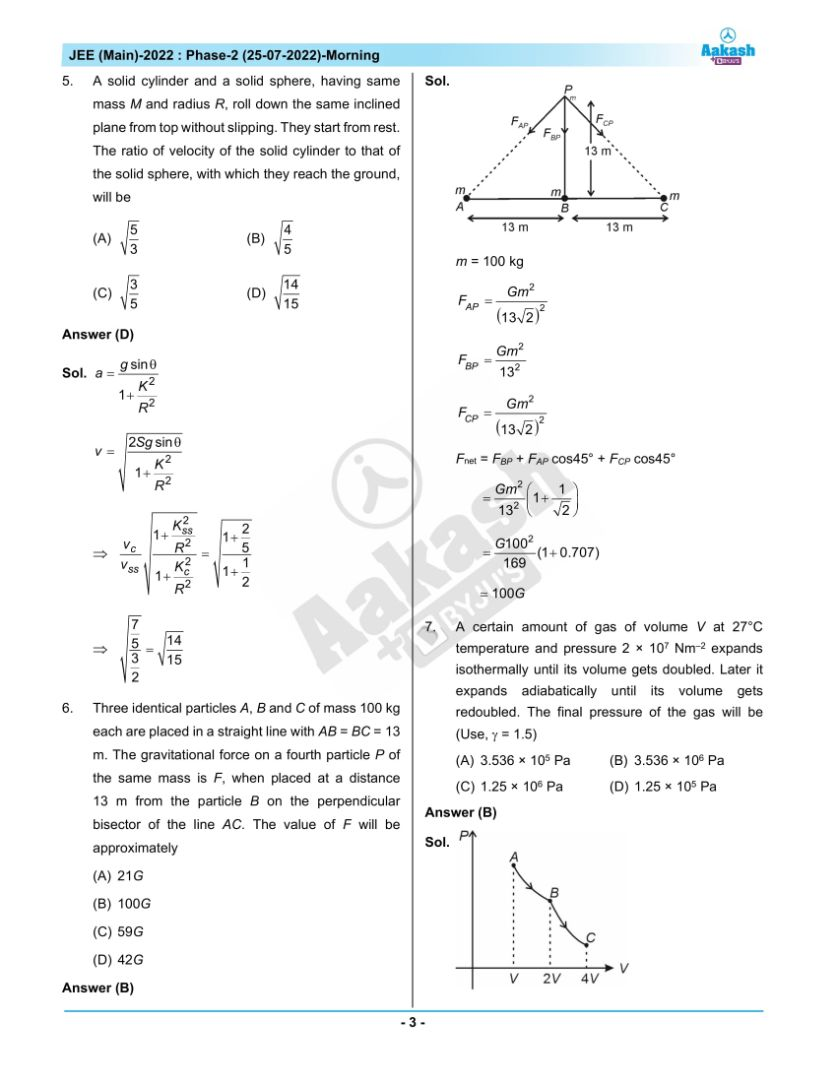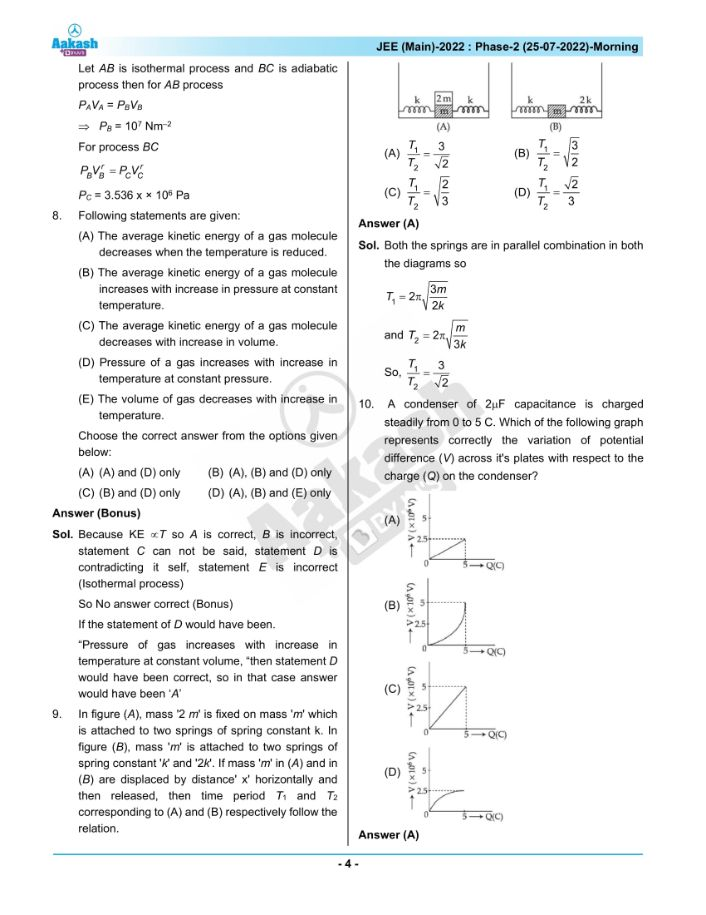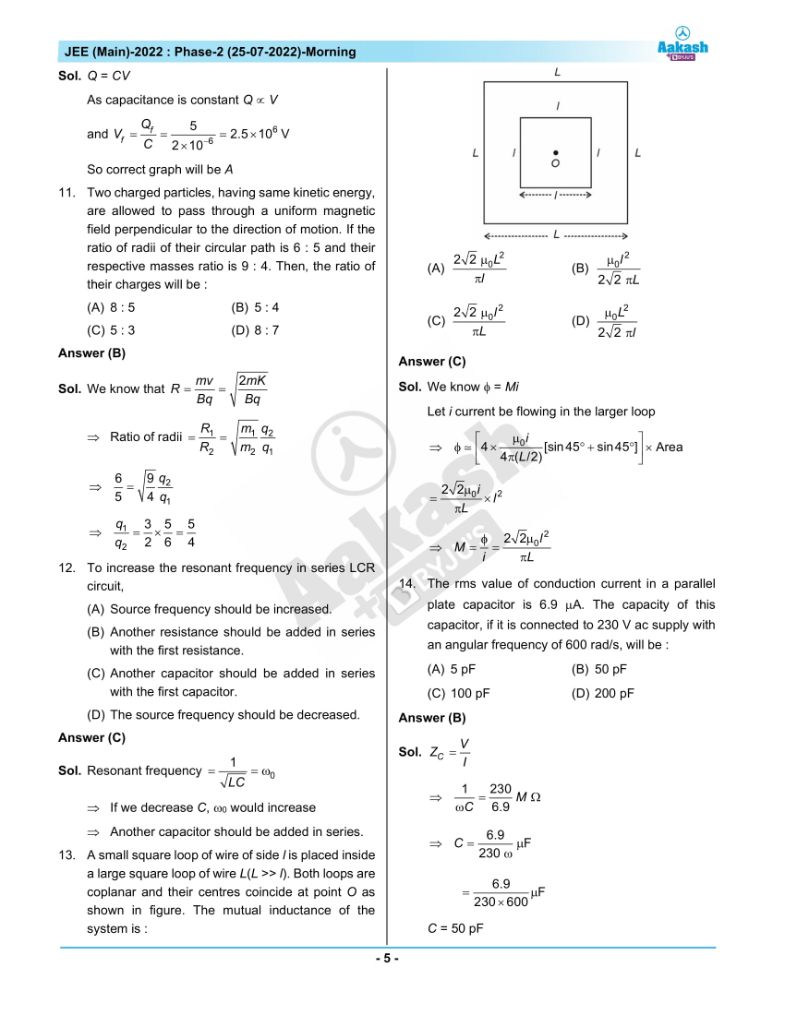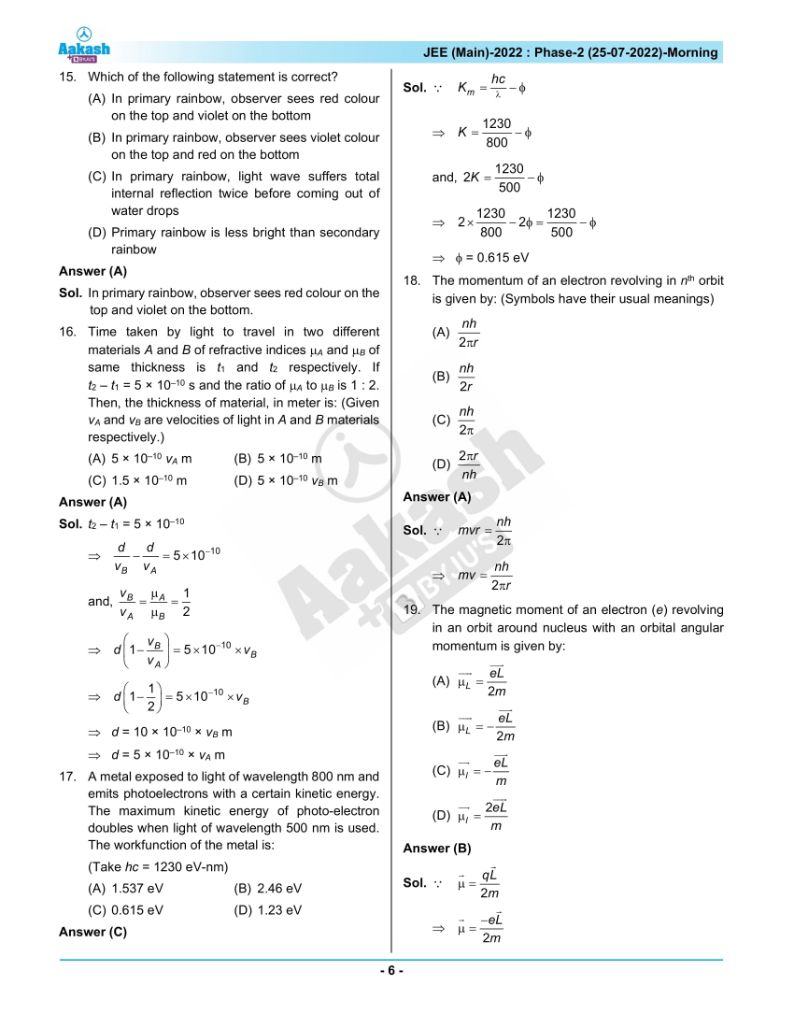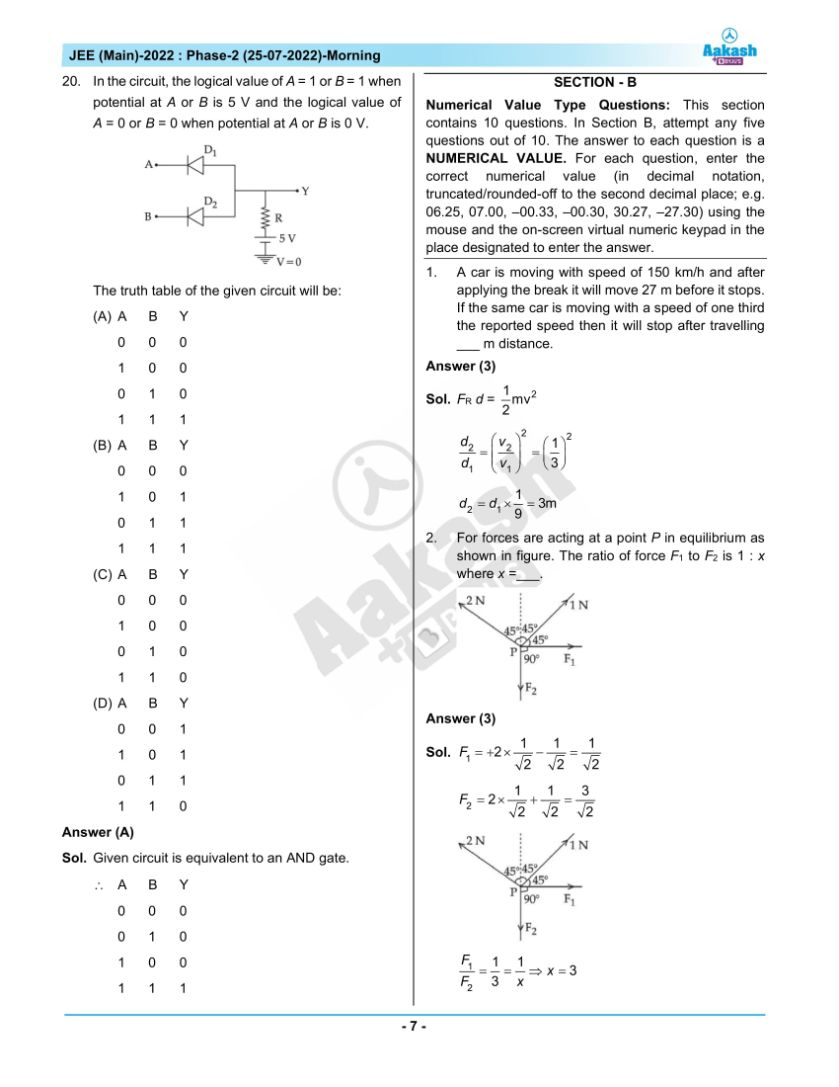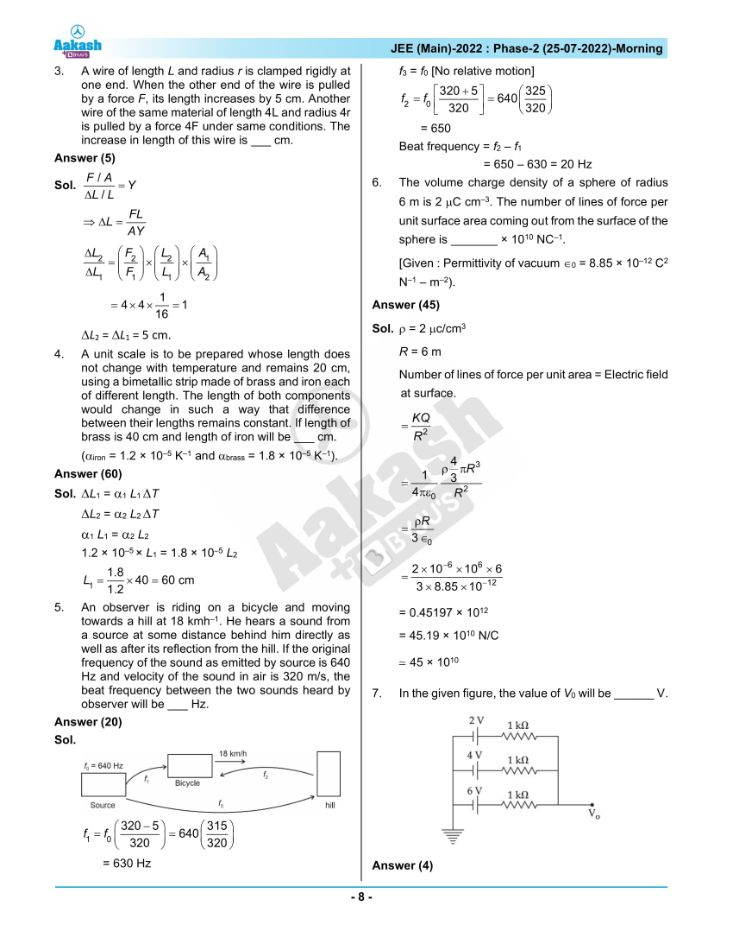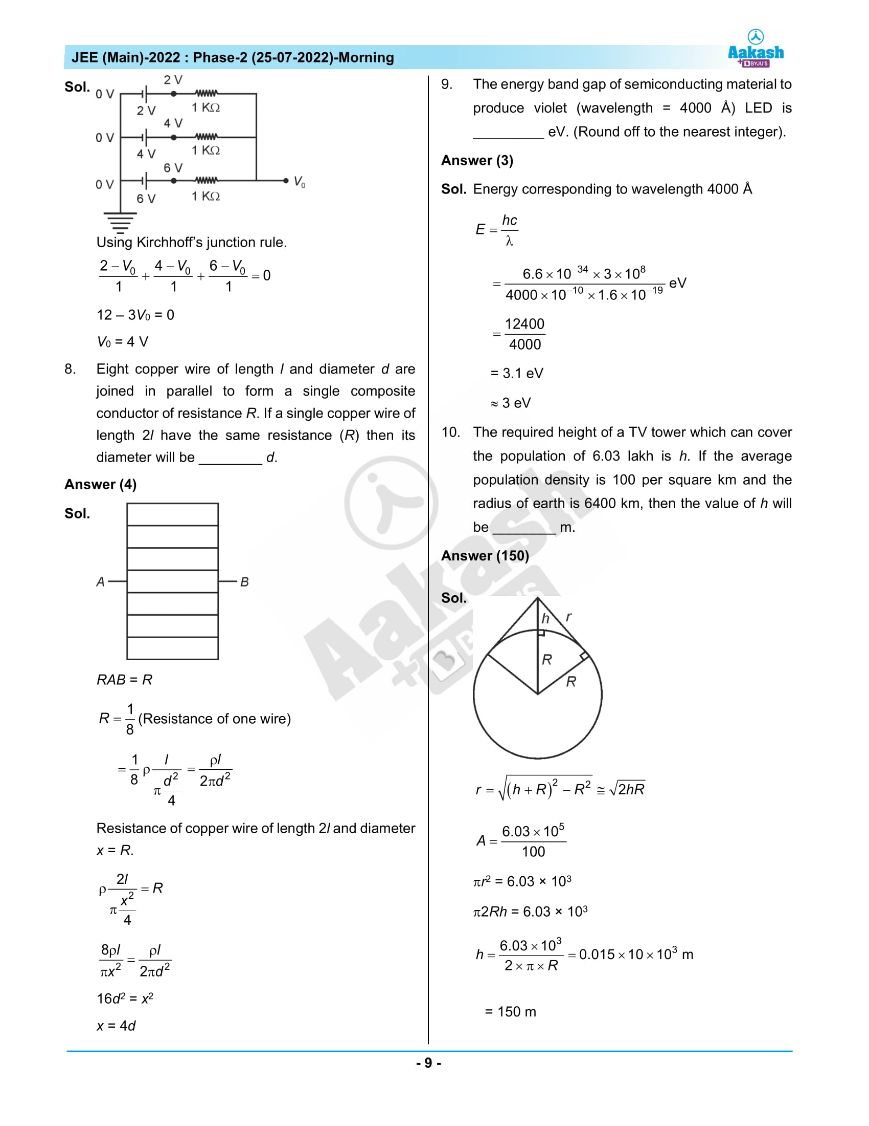## JEE Main 2022 July 25th Shift 1 Paper Analysis